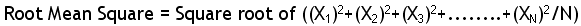Home Math Calculators Math  Flashcards Math Games Math  Homework Math Lessons Math Terms Math Worksheets Math Tools
You Are In Math Glossary of Terms

# Root Mean Square

It is Square root of the mean of square of value of variable.

It is a statistical measure of the magnitude of variation.where

X = Individual values

N = Sample size

Example:

Find Root Mean Square of 5, 6, 8

We first calculate mean (average) of square of values. = ( 25 + 36 + 64) / 3 = 41.67

Therefore, Square root of 41.67

or 6.46